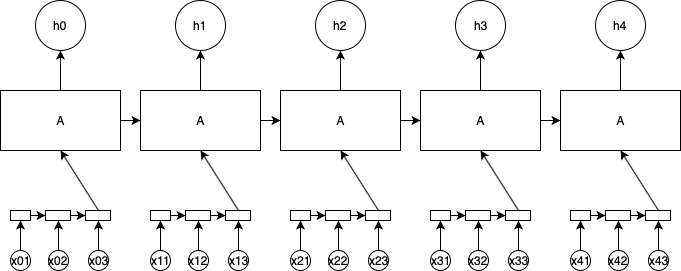# LSTM stock market risk

#1

HiI’m currently on a project, where I want to use an LSTM to predict the risk (in terms of volatility/standard deviation) of stock price returns for the next day. I got intra-day trading data and I’m trying to find the best input shape for the model.

My idea is as follows: Having the intra-day trades I calculate the log-returns of each trade and calculate the standard deviation for each 5 minute interval. As there are 6.5 trading hours per days, this gives me 78 time steps. From this the standard deviation of the returns of the next day shall be predicted (so there is 1 value for y).
What I first did, in an array of shape (days, 78, 1), e.g. if I have 400 days in my training set, it would be (400, 78, 1), so there are 400 samples of 78 time steps of 1 feature to predict the next days volatility.

In my understanding this does not really do, what I want. As it takes 400 samples, the state of last days prediction is not encountered for the next day. It only takes the intra-day 78 time steps and predicts the next days value. After that it takes the next sample.

What I would like to do is:
1.) Taking the 78 intra-day time steps to predict a hidden layer
2.) Using this prediction of, say, the last 7 days to predict the volatility of the next day

like:
model = tf.keras.models.Sequential()
model.add(tf.keras.layers.LSTM(32, input_shape(78, 1))) -> output shape (1,)
model.add(tf.keras.layers.LSTM(16, input_shape(7,1)))
model.add(tf.keras.layers.Dense(1, activation=‘relu’))

but this, of course does not work, because the output of the first LSTM does not have the 7 time steps but one, because it is only calculated for one day, not for the last 7.

Instead of only using the intra-day state, the model should also use the state of the last realizations of the volatility.

Do you have an idea how to implement this or am I completely on the wrong track?

EDIT: Maybe it is easier to understand when I add a graph:0 Likes

(Malcolm McLean) #2

Hi. Welcome to the forum and thanks for posting your question. I found your post because I also have a casual interest in stock market prediction.

First, (in case you have not realized it by now) fastai is built on PyTorch. Though Keras was used in much earlier versions, most forum users are focused on using fastai, PyTorch, and most recently Swift/Tensorflow. Therefore you may have better luck posting in a community that actively uses Keras and Tensorflow.

A couple of suggestions: there are several fastai lessons that address RNNs. Time series are not specifically addressed (the focus is on language). However, studying them would fill in the big picture about RNNs and clarify some of your confusions around designing an appropriate model.

Fortunately, there is also a thread specifically devoted to time series. There you are more likely to find help with specific questions.

HTH, and good luck with your project!

0 Likes